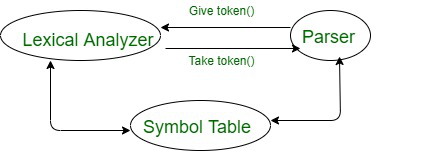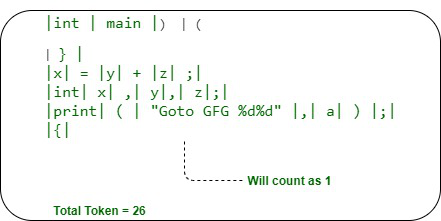# Working of Lexical Analyzer in compiler

• Difficulty Level : Basic
• Last Updated : 26 Aug, 2020

In this article, we are going to cover how the lexical analyzer works and will also cover the basic architecture of lexical analyzer. Let’s discuss one by one.

Pre-requisiteIntroduction to Lexical Analyzer

Lexical Analyzer :

• It is the first phase of a compiler is known as Scanner (It’s scan the program).
• Lexical Analyzer will divide the program into some meaningful strings which are known as a token.Types of token as following –

1. Identifier
2. Keyword
3. Operator
4. Constants
5. Special symbol(@, \$, #)

Above is the terminologies of token which is the key component for working in Lexical Analyzer. Now, with the help of example, you will see how it works.

Let’s consider the following C program given below to understands the working.

```int main)(
}
x = y+z;
int x, y, z;
print("Goto GFG %d%d", a);
{ ```

In the first phase, the compiler doesn’t check the syntax. So, here this program as input to the lexical analyzer and convert it into the tokens. So, tokenization is one of the important functioning of lexical analyzer.

The total number of token for this program is 26. Below given is the diagram of how it will count the token.In this above diagram, you can check and count the number of tokens and can understand how tokenization works in lexical analyzer phase.

This is how you can understand each phase in compiler with clarity and will get an idea of how compiler works internally and each phase of the compiler is the key step.

My Personal Notes arrow_drop_up